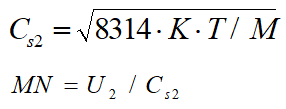Gas, Steam - Intergraph Smart Instrumentation - 13.1 - Reference - Hexagon PPM

Integraph Smart Instrumentation Sizing Equations

Language
English (United States)
Product
Intergraph Smart Instrumentation
Search by Category
Reference
Smart Instrumentation Version
13.1
1. Calculate XT: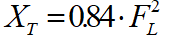2. Calculate effective velocity head coefficients: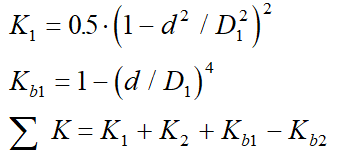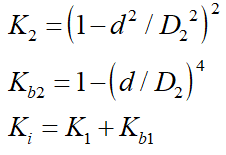3. Calculate FK: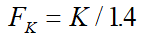4. Calculate X: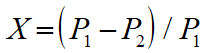5. Calculate first value of CV:

*d [in]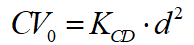6. Calculate FP: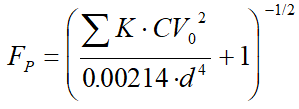7. Calculate XTP: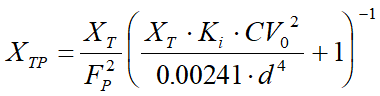8. The condition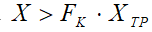determins:

Case A - Critical flow

1. Eps=0.667.

2. Calculate new CV: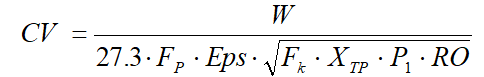Case B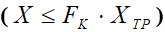- Usual

3.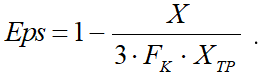4. Calculate new CV: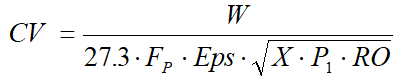9. Calculate the relative change in CV: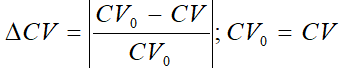10. Steps 6 to 9 are repeated until the relative change in CV is less than 0.001.

11. Calculate outlet pipe velocity: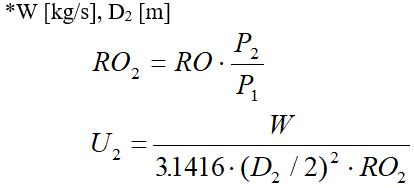12. Calculate outlet Max number: